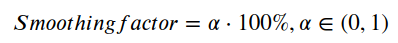# Auto optimization

## Offer auto-optimization

The performance of the certain offer can dynamically change in the split test during the day.
We wanted to relieve you of the necessity of changing the weights by hand and so we've come up with an Offer optimization setting. Once you enable it, the weights of all the offers will update automatically according to the algorithm described below.
In order for the algorithm to work as expected, you are not to use more than 10 offers in one Path. If you have more than 10 offers, you can split them between several Paths.
The weights are calculated in several steps. We strongly recommend that you have a look at the formulas and the example below before you use the advanced settings in order to understand the logic behind the algorithm.
Please note, auto-optimization works only with type of distribution: Normal rotation.

## The amount of conversions forecast

It is necessary to foretell the amount of conversions for the next time frame to determine the weight of the offer
Formula: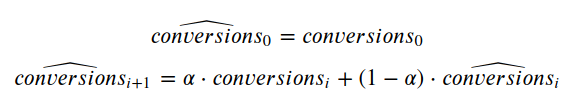where:

•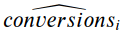- the foretold amount of conversions for the i time frame
•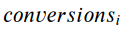- the real amount of conversions for the i time frame
•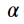- smoothing factor (from 0 to 1)

## Calculation of the offer ratio

The following formula is used as an offer ratio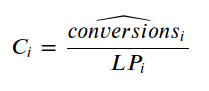where:

•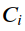- the efficiency ratio of the offer for the i time frame
•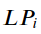- the real amount of all LP clicks for the i time frame

## Calculation of the weight

The new weight is it's part of the ration in the sum of all ratios in the Path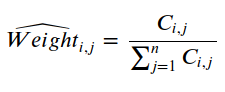There is a threshold of 5% in order to avoid the scenario when the algorithm gives an offer very little weight or disables it, so the offer will never go below 5%.
Because of the threshold from the previous step, the sum of the weights in the Path might not be equal to 100%. This is where the adjustment kicks in.
Let's first define high and low weights: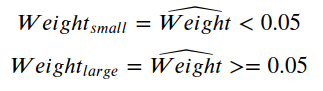Low weights are amounted to the lowest threshold: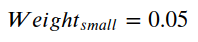Calculating the reduction ratio for high weights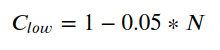where:

•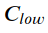- reduction ratio,
•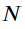- the amount of all weights

Adding the arithmetic mean of the low weights to the high weights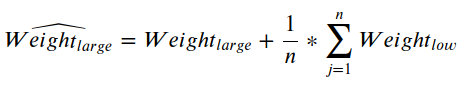where:

•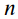- the amount of low weights

Multiplying the new high weight by the reduction ratio and add a lower threshold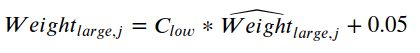where:

•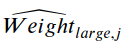- new high weight of the j offer from the previous step
•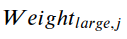- adjusted high weight of the j offer